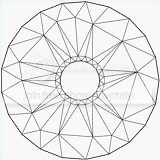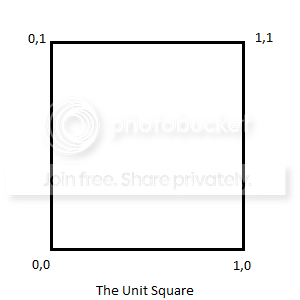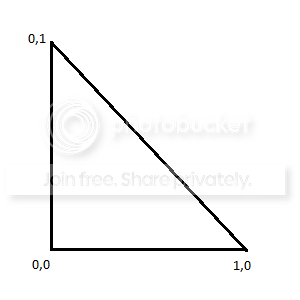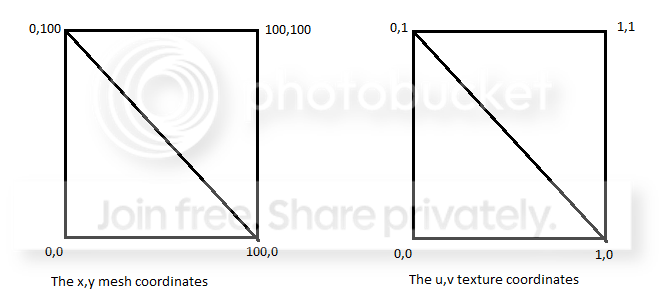#### Howdy, Stranger!

It looks like you're new here. If you want to get involved, click one of these buttons!

# Mesh Tutorial

edited June 2012 in General Posts: 179

Hello, after I had a little trouble grasping the concepts of how meshes work and how to texture them (and subsequently receiving help from @Andrew_Stacey and @Shrike) I decided to create a tutorial on the basics of meshes. If you have time, please read it over and see where I have failed to explain things clearly, or where I give bad info. Once I get corrections made, I will add it to the wiki:

Mesh tutorial

In this tutorial, I am going to talk about what meshes are, why you might want to use them, and how to use them in Codea. To complete this tutorial, you probably want to have a basic understanding of Codea and the LUA language. Let's call the level of difficulty "intermediate."

What Meshes Are

First off, let's talk about what a mesh is. You might think of a mesh as a 3D object, and meshes ARE a basic part of 3D objects. But a mesh could more accurately be defined as as a list of polygons, and more specifically, in the case of Codea, it is a list of triangles. These lists of polygons are very useful for both 2d and 3d game creation.

Basically any 2d or 3d shape can be broken up into a bunch of triangles. Generally, the more polygons used, the smoother the shape.In modern 3d games, the models are composed of thousands (or tens of thousands) of polygons. To accomplish this feat, the iPad graphics hardware has been designed to manipulate and draw triangles extremely fast, much faster than just drawing pixels to the screen. By using meshes in Codea rather than using the sprite command, you can often drastically speed up the rendering in your game.

To create a mesh you simply run the built in mesh function, which returns an empty mesh.

``````myMesh = mesh()
``````

That mesh is pretty worthless without any triangles, so let's add a triangle to it. Here is a diagram of the first mesh we are going to create:And here is the code to add that triangle to our mesh:

``````myMesh.vertices = {vec2(0,0),vec2(100,0),vec2(0,100)}
``````

And now we have a functional mesh. Let's make a quick program to test it out:

``````function setup()
myMesh = mesh()
myMesh.vertices = {vec2(0,0),vec2(100,0),vec2(0,100)}
end

function draw()
background(40, 40, 50)
myMesh:draw()
end
``````

Run this and you will see our grey triangle appear in the bottom left hand corner of the screen at the origin. The triangle is rendered in grey because we have not given it any color or texture. Let's try giving it color first, and we will graduate to textures later.

Coloring Meshes

To add color to a mesh you pass a list of colors to the mesh that is equal in length to the number of vertices. This means that for every vertex in the list, you must provide a color. Our mesh currently has 1 triangle and therefore 3 vertices, so we need to give the mesh 3 colors. Here is the updated program with colors added:

``````function setup()
red = color(255, 0, 0, 255)
green = color(0, 255, 0, 255)
blue = color(0, 0, 255, 255)

myMesh = mesh()
myMesh.vertices = {vec2(0,0),vec2(100,0),vec2(0,100)}
myMesh.colors = {red, green, blue}
end

function draw()
background(40, 40, 50)
myMesh:draw()
end
``````

Now our mesh is rendered in color, and we get a pretty neat gradient effect as the colors are blended between the vertices. You can use this technique to create gradient backgrounds, buttons, etc.

Texturing Meshes

Texturing meshes works a lot like coloring meshes, but instead of passing a list of colors, we will pass a list of texture coordinates. This is a process called U,V mapping. The x,y coordinate of each vertex is mapped to a coordinate on the texture, which is called the u,v coordinate, since x and y are already in use. (Hence the name U,V mapping) With the U,V coordinates, we use a "unit-square" system, which allows us to specify what portion of the texture we want to use for the triangle, instead of the exact pixels. (Don't worry, this will be explained more clearly as you follow this example.)

This is a diagram of the unit square:If you want to use the full width of the image, you would use 1 as the width. To use half of the width, you would use .50 as the width. Let's take an image from the Codea spritepacks and use it as our texture. First get rid of the color code as we are now texturing instead. Next we want to make a triangle in U,V coordinates that matches our mesh triangle. It will look something like this:So the texture coordinates for our triangle are going to be:
0,0 1,0 0,1
Can you see how those relate to our vertices? Remember they are:
0,0 100,0 0,100

Here is the updated code:

``````function setup()
img = readImage("Planet Cute:Icon") --Get the image

myMesh = mesh()
myMesh.vertices = {vec2(0,0),vec2(100,0),vec2(0,100)}
myMesh.texture = img --Set the image as texture
myMesh.texCoords = {vec2(0,0),vec2(1,0),vec2(0,1)}
end

function draw()
background(40, 40, 50)
myMesh:draw()
end
``````

And if done correctly, we should now have half of the image drawn on our triangle. To see the rest of this image, I would really like to have another triangle to finish the rectangle. So, looking at this image:You should see that the triangle we need to add to our mesh has the vertices: 0,100 100,100 100,0 And the texture coordinatesfor that new triangle will be: 0,1 1,1 1,0. Lets add them to our code:

``````function setup()
img = readImage("Planet Cute:Icon") --Get the image

myMesh = mesh()
myMesh.vertices = {vec2(0,0),vec2(100,0),vec2(0,100),vec2(0,100),vec2(100,100),vec2(100,0)}
myMesh.texture = img --Set the image as texture
myMesh.texCoords = {vec2(0,0),vec2(1,0),vec2(0,1),vec2(0,1),vec2(1,1),vec2(1,0)}
end

function draw()
background(40, 40, 50)
myMesh:draw()
end
``````

And Voila, we have our image drawn correctly to a mesh. Now that we have learned how to draw a rectangular texture onto a mesh the hard way, lets look at a slightly simplified way of completing that same task.

Tagged:

• Posts: 179

The Rectangle Functions

It is very common to want to use rectangles in your meshes like we did above. To make it easier on us, Codea has a mesh function called addRect. addRect automatically adds two right(angle) triangles to your mesh that together make a rectangle. It can also automatically create the proper texture coordinates. To replicate the work we did above, we could use:

``````myMesh:addRect(50,50,100,100)
``````

Much easier, huh? The reason we used 50,50 there instead of 0,0 is because the addRect function creates the mesh CENTERED on the x,y coordinate given.

So the following code could do what we did above:

``````function setup()
myMesh = mesh()
myMesh.texture = img
end

function draw()
background(40, 40, 50)
myMesh:draw()
end
``````

You can also adjust the texture on rectangles. This is especially useful if you want a mesh with one texture stretched over a grid of rectangles. When you create a new rectangle using addRect, the function will return a unique identifier for that rectangle that can be used to modify the texturing (u,v mapping) of the rectangle. You do it like this:

``````idx = myMesh:addRect(50,50,100,100)
myMesh:setRectTex(idx, 0, 0, 1, 1)
``````

The arguments you pass to the setRectTex function are:
(index, u, v, width, height)

So if I want to only use the bottom-left 1/4th of the image as my texture I would do so like this:

``````myMesh:setRectTex(idx,0,0,.50,.50)
``````

And if I wanted to use the top left 1/4 of the image I would use:

``````myMesh:setRectTex(idx,0,.50,.50,.50)
``````

When creating rectangular meshes, it is generally much easier to use the rectangle functions. If you are just using meshes to draw sprites quickly on the screen, then using addRect will likely be much more user-friendly than creating a list of vertices and creating a complimentary texture coordinate list.

To be continued...

In a future tutorial I will cover moving meshes into 3d and using indexed colors. If there are other topics concerning meshes you would like me to explain, please let me know.

• edited June 2012 Posts: 121

Hi, great job @Vega.

One more important thing about addRect and other rect functionalities: addRect is just a short way to add 6 vertex (so 2 triangles).

If you use addRect to create your mesh, the return value is nothing more then a progressive value and you can use it also to index specific vertex, knowing that rect with idx = i has vertices with idx from (i-1)6 + 1 to (i-1)6 + 6

I hope to remember right (please have a test...) that the rect should be formed in this way:

``````v1=v4-- v6
|   \      |
|     \    |
|       \  |
v2----v3=V5
``````

Moreover pay attention because if you first set directly the vertex buffer (like in the beginning of your tutorial) and then you call an addRect, the vertex buffer gets cleared and reset, so the addRect set the first 6 vertices and returns 1 as rect idx (or at least is what it seems to me after some try, maybe better to ask to @Simeon or @Andrew_Stacey about that!)

Last thing: you can't remove vertices from a mesh, if you really need that, the only way is to clear and set a new vertex buffer.

• Posts: 563

Brilliant - fantastic tutorial @Vega.

• Posts: 2,161

Didn't spot anything wrong. Some ideas for expansion (trying to keep to the same level):

1. You can set a uniform colour all in one go.
2. Order matters - experiment to be sure, but I think the texture has to be set before the texture coords, and a global colour only affects previously declared coords.
3. You can set colours and textures, in which case the colours tint the texture.
• edited June 2012 Posts: 5,705

Edit: I've added links to the WIki and Issue Tracker at the top of the forums. Hopefully they will be more accessed now.

Amazing post, @Vega. Thank you for sharing what you learned. I second adding it to the wiki.

You can't remove vertices from a mesh, but you can effectively remove triangles by collapsing their vertices — they will still be "in" the mesh, but won't be rendered. If you plan to add and remove a lot of triangles (for example, in a particle system), it's best to allocate a whole bunch of vertices and then arrange them into triangles when you need them, and collapse them when you don't.

(Vertices are cheap to pass to the GPU, so this is more cost effective than re-allocating your mesh all the time.)

• Posts: 179

Thank you for the feedback. I will add a few things and revise a bit and post it to the wiki. I will continue spelling it color, however, as I am from USA. Sorry, people from the rest of the world.

• Posts: 384

This is extremely well-written, @Vega! You've made sense of it for me, and this will be a great addition to the wiki.

• Posts: 5,705

@Vega color is good. I'd rather keep everything consistent with US spelling where possible. Especially as that's the name of the type.

• Posts: 179

Finally got it added to the Wiki in the tutorials section just below the tutorial on vec2. Not sure if that is the best place, feel free to move it if you like.

• Posts: 11

Thank you very much, Vega. I finally can try to mess with mesh.
Played with it a lil bit and got the following that lets you add a triangle to a mesh every three points you touch... Kinda... (altho in between it does some weird stuff...)

``````function setup()
tap = false
m = mesh()
pt1 = vec2(0,0)
pt2 = vec2(0,0)
pt3 = vec2(0,0)
end

function draw()
if CurrentTouch.state == BEGAN and not tap then
tap = true
end
if tap then
if CurrentTouch.state == ENDED then
pt1 = pt2
pt2 = pt3
pt3 = vec2(CurrentTouch.x, CurrentTouch.y)

m:vertex(i, pt3)
m:color(i, math.random(255), math.random(255), math.random(255), math.random(255))

tap = false

end
end

background(53, 53, 53, 255)
m:draw()
fill(231, 231, 41, 255)
ellipse(pt1.x, pt1.y, 20)
ellipse(pt2.x, pt2.y, 20)
ellipse(pt3.x, pt3.y, 20)

end
``````

%%-

• Posts: 381

Great tutorial, @vega. Suggestion: please add screenshot for every given example. That way, the learners would know whether his/her code is correct or not. Thank you.

Looking forward for the advance chapters.• Posts: 179

That seems to work well, @Toshio. You may have noticed that there is currently no function for addTriangle, which I believe would be a great idea for a future addition. (@Simeon, what do you think?)

To add a triangle you would code something like this:

``````verts = myMesh.vertices
table.insert(verts, newVec2a)
table.insert(verts, newVec2b)
table.insert(verts, newVec2c)
myMesh:clear()
myMesh.vertices = verts
``````

That is a little slow however, because you are clearing and recreating the mesh each time you add a triangle.

• Posts: 580

Not sure a function to create a triangle would actually be all that useful. For a rect it's great, because you only need to specify x, y, w, and h, but there's really no getting around the fact that you need to specify 3 points for a triangle.

• Posts: 5,705

@Vega we thought about it, but as @toadkick mentioned, you would need up to 9 arguments to specify the triangle's vertices. I felt that this was more neatly done over multiple lines. In addition, you would not be able to use addTriangle and addRect reliably together - as addTriangle would break the returned rect indices.

Still I agree in principle that there needs to be a better way to add triangles.

• edited June 2012 Posts: 489

Thank you @Vega for a very helpful guide.

Drawing the two meshes side by side - the two triangles (`myMesh1`) and the rectangle (`myMesh2`) - I noticed that the first was dull and the second bright. Picking up what @Andrew_Stacey explains about colours tinting textures, it seems to me that a default grey tints one mesh, but not the other. (Adding `myMesh1:setColors(255, 255, 255, 255)` after the vertices have been set clears the dullness.)

• Posts: 489

The `.vertices` syntax for the `mesh()` userdata follows the Lua syntax for setting a field of a table:

``````vertexTable = { --[[ table of vec2 or vec3 --]] }
m = mesh()
m["vertices"] = vertexTable
``````

I am correct to understand, however, it is more akin to a function (`m:vertices(vertexTable)`), because to change the vertices you cannot simply update the table referenced (in my example) by `vertexTable`)?

• Posts: 179

I'm not sure exactly how it works, but it seems you do have to set the whole table at once. In my. experiments I tried to change a single vertex like

``````m["vertices"] = vec2(200,200)
``````

And that does not work. You must set the entire vertices table at once. So you would have to do

``````vertexTable = vec2(200,200)
m["vertices"] = vertexTable
``````

Maybe I should take the time to look at the API to see how it really works.

• edited June 2012 Posts: 580

mesh has a method called vertex() that allows you to set/get the position of a vertex. For example,

``````myMesh:vertex(1, 100, 100)
``````

will set vertex 1's position to (100,100). Calling it with just the index will return the vertex's position at that index as a vec3. Now, what I'm not sure of at the moment is whether or not you can do that without first adding the verts by assigning them to the .vertices field or by using addRect, but I do know for sure that once the verts are there they can be modified using the vertex() method. There are also color() and texCoord() methods that allow you to modify/get the color/texture coordinates in the same way

• Posts: 489

The `m:vertex(index, ...)` function reports an error if the index is out of bounds. I was considering changing metatables to get a different behaviour, starting with this experiment:

``````-- Rewire the functionality...
local mmt = getmetatable(mesh())
local oldmeshnewindex = mmt["__newindex"]
mmt["__newindex"] = function(m1, k1, v1)
if k1=="vertices" then
local vmt1 = getmetatable(v1) or {}
local oldv1newindex = vmt1["__newindex"] or rawset
vmt1["__newindex"] = function (t2, k2, v2)
oldv1newindex(t2, k2, v2)
m1.clear()
m1.vertices = t2
end
setmetatable(v1, vmt1)
end
oldmeshnewindex(m1, k1, v1)
return
end

-- Example of the rewired mesh userdata
function setup()
m = mesh()
vertexTable = {vec2(0,0), vec2(100,0)}
m.vertices = vertexTable
print("Size of mesh:", m.size) -- Outputs 2

vertexTable[#vertexTable + 1] = vec2(0, 100) -- But table.insert() does not work...

print("New size of mesh:", m.size) -- Outputs 3
end

function draw()
background(40, 40, 50)
end
``````

but it would need more code to avoid changes to the table forever affecting the mesh userdata that it has now become associated with.

• edited June 2012 Posts: 580

@mpilgrem: that's what I figured, and it makes sense. table.insert() won't work because under the hood I'm sure that mesh just uses a straightforward memory buffer (don't have the code in front of me at the moment, but I'll bet it's a std::vector). It might be cool to have an addVertex() method to tack on a vertex to the end of the existing buffer, and/or an insertVertex() method that would shift higher indexed vertices up (though that would be a bit expensive). Dunno, I'll have to think about it some more. Not sure that there are enough common use cases to warrant the API additions.

• Posts: 489

Continuing my experiments in how Lua can be used to rewire built-in functionality, the following code rewires `rawset`, `table.insert` and the `mesh` userdata to make `m.vertices = vertexTable` behave like its syntax implies - linking the table with the mesh userdata.

``````-- Rewire rawset()
local oldrawset = rawset
rawset = function (t, k, v)
local r = oldrawset(t, k, v)
local mt = getmetatable(t) or {}
-- Is the table linked to meshes?
if mm then
-- Update each mesh
for _, m in pairs(mm) do
m.clear()
m.vertices = t
end
end
return r
end

-- Rewire table.insert()
local oldtableinsert = table.insert
table["insert"] = function (t, a1, a2)
if a2 then
oldtableinsert(t, a1, a2)
else
oldtableinsert(t, a1)
end
local mt = getmetatable(t) or {}
-- Is the table linked to meshes?
if mm then
-- Update each mesh
for _, m in pairs(mm) do
m.clear()
m.vertices = t
end
end
end

-- Rewire __newindex of mesh userdata
local mmt = getmetatable(mesh())
local oldmeshnewindex = mmt["__newindex"]
mmt["__newindex"] = function(m1, k1, v1)
if k1=="vertices" then
-- Is a vertex table linked to mesh?
if VT then
-- Clear the table's link to the mesh
local VTmt = getmetatable(VT)
end
-- Link the vertex table to the mesh
local vmt1 = getmetatable(v1) or {}
vmt1["__newindex"] = vmt1["__newindex"] or rawset
setmetatable(v1, vmt1)
end
oldmeshnewindex(m1, k1, v1)
return
end

-- Example of use
function setup()
m1 = mesh()
m2 = mesh()
vertexTable1 = {vec2(0,0), vec2(100,0), vec2(0, 100)}
-- Link one vertex table to two meshes
m1.vertices = vertexTable1
m2.vertices = vertexTable1
print("Size of mesh 1:", m1.size) -- Outputs 3
print("Size of mesh 2:", m2.size) -- Outputs 3

vertexTable1[#vertexTable1 + 1] = vec2(0, 100)
vertexTable1[#vertexTable1 + 1] = vec2(100, 100)
vertexTable1[#vertexTable1 + 1] = vec2(100, 0)
print("New size of mesh 1:", m1.size) -- Outputs 6
print("New size of mesh 2:", m2.size) -- Outputs 6

vertexTable2 = {}
-- Link a new vertex table to one of the meshes
m1.vertices = vertexTable2
table.insert(vertexTable2, vec2(100, 0))
table.insert(vertexTable2, vec2(200, 0))
table.insert(vertexTable2, vec2(100, 100))
print("Final size of mesh 1:", m1.size) -- Outputs 3
print("Final size of mesh 2:", m2.size) -- Outputs 6
end

function draw()
background(40, 40, 50)
end
``````
• Posts: 5,705

@mpilgrem from a glance at the code, that will clear the mesh every time the vertex table is modified? I think it's a great piece of code — especially if you use it to set up a fairly static mesh. But for dynamic meshes you might not want to do that (if they get large).

• Posts: 489

@Simeon, you're absolutely right, of course. I should have made clear that - currently - extending a general mesh userdata's vertices, once they have been set initially, will involve an inefficient 'reset', no matter what syntactic approach is adopted. I was experimenting with syntax, really - rather than proposing something useful for dynamic situations.

• Posts: 643

Looks interesting. What you could do is have a flag that is set when you modify the table, and alter mesh's draw function to copy the vertices over and reset the flag before drawing.

• Posts: 489

I have added this page to the wiki. My aim was to provide reference-type material to complement @Vega's tutorial, and add to what is set out in the in-app reference.

• Posts: 179

Good info there, @mpilgrem. I wish I had that when I was learning about Meshes.

• Posts: 115

Nice work @Vega and @mpilgrem.

Learning is getting easier with everyone's help.

Thanks a lot.

• Posts: 3

Update - the Wiki mesh page by mpilgrem moved to https://bitbucket.org/TwoLivesLeft/core/wiki/mesh

(added this update in case anyone thought the page had been deleted as the link above doesn't work).

Vega's tutorial is at https://bitbucket.org/TwoLivesLeft/core/wiki/mesha

thanks for the tips, folks!